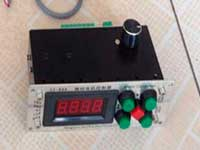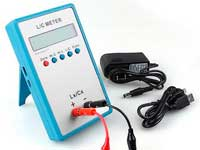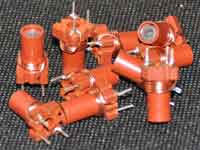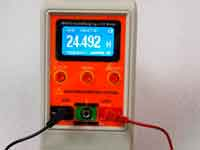Helical resonator bandpass filter - 3.8 out of 5 based on 4 votes
Rating 3.75 (4 Votes)

Helical resonator bandpass filterSpiral resonator - is a coaxial trasmission line with the inner conductor rolled into a helix. This is the correct definition. On the other hand it can be seen as a high-Q shielded inductor that operates at a self-resonance frequency. The shield introduces additive in distributed capacitance of the coil and reduce its resonant frequency. Despite the fact that the filter on the spiral resonators difficult to compete with SAW-filters, especially in terms of sizes, interest to its has not diminished since. They allow radio-enthusiasts to create a linear narrowband filter.

Spiral resonator has invented by Alexander Horvath in 1950, but the first method of calculation was published in 1967 in the book "Handbook of Filter Synthesis" by A.I.Zverev. We will view it here very briefly with adaptation to metric measurement units. See more in the original book.

Before we consider this method, you need to understand one very important point. Inductance and self-capacitance of the coil are determined at a relatively low frequency, far below its self-resonance. The current density in all turns is considered to be the same and these coil parameters depend only on its winding geometry. However, at frequencies close to self-resonance we are beginning to see the wave effects in the helix. In this case the current density in all turns can not be considered the same. The values of inductance and self-capacitance begin to "float" by frequency. In the end, "low-frequency" classic formulas no longer work and we can't get them to correctly calculate the helical resonator. Now the computer modeling can help us. And in the middle of the XX century had "predictor-corrector method" to explore helical resonators, and to find the formula for its calculation. What actually is the Zverev's method.

In fact, the system of Zverev's equations is the optimal empirical description of a spiral resonator with the geometric relationships that got experimentally to maximize the quality factorFor a resonator with circular form of the shield the ratio as follows:
The name of parameterFormulaunitsN
The ratio of the winding diameter (d) to the shield diameter (D)  d/D = 0.55 cm [1.1]
The distance from the helix to the top and bottom of the shield (G) G = 0.25D  cm [1.2]
The ratio of shield height (H) to shield diameter (D) H/D = 1.5  cm [1.3]
For a resonator with a square screen (the shield side S = D / 1.2) the following ratio:
The name of parameterFormulaUnitsN
The ratio of the winding diameter (d) to the shield side (S)  d/S = 0.66 cm [2.1]
The distance from the helix to the top and bottom of the shield (G) G = 0.3S  cm [2.2]
The ratio of shield height (H) to shield diameter (S) H/S = 1.6  cm [2.3]
General formulas for the spiral resonator:
The name of parameterFormulaUnitsN
The ratio of the helix length (b) to the winding diameter (d)  b/d = 1.5  cm [3.1]
The thickness of the skin layer δ δ = 6.6⋅10-3/√f0  cm, MHz [3.2]
Winding pitch τ = b/N  cm [3.3]
Wire diameter (d0):  d0 = 0.5τ;  d0 > 5δ cm [3.4]
Number of turns of the helix (N) N = 2674/[f0d ]  cm, MHz [3.5]
The quality factor of the resonator Qu = 35.9d√f0  cm, MHz [3.6]
The characteristic impedance of resonator Z0 Z0 =  136190/[f0d] Ohm, MHz, cm [3.7]
The dependence of the quality factor Qu on the volume of the resonator V Qu = 20√f0?V  cm, MHz

[3.8]

Calculation of helical resonators filter

Calculation of helical resonators filter with an arbitrary number of element is quite complicated. We can simplify the task and calculate 2-elements filter. The calculation will be carried out step by step on example..1. Initial data for calculation:
• Working frequency - f0
• Bandwidth on level -3 dB - ΔF
• Admissible loss in the passband - α
• Input and output impedance - Ri, Ru

Suppose we have the following data: f0 = 100MHz; ΔF = 1МГц; Ri = Ru = 50 Ohm; α = 1 dB
2. We define the unloaded Q of the resonator Qu
 Qu = (f0/ΔF)⋅Q0 Q0 = 1.414/(10α/20 - 1) [4.1]
Q0 = 1.414/(101/20 - 1) = 11.59;
Qu = (100/1)⋅9.35 = 1159;
3. The formulas [3.1] and [3.6] define the size of the helix:
d = Qu / (35.9√f0) = 935 / (35.9⋅√100) = 1159 / 359 = 3.2 cm;
b = 1.5d = 1.5⋅3.2 = 4.8 cm;
4. The formulas [3.3], [3.4], [3.5] define the number of turns, winding pitch and diameter of the wire:
N = 2674 / (100⋅3.2) = 8.3 turns;
τ = 4.8 / 8.3 = 5.8 mm;
d0 = 5.8 / 2 = 2.9 mm;
5. The formulas [2.1], [2.3] define the size of the shield:
S = 3.2 / 0.66 = 4.9 cm;
H = 4.9⋅1.6 = 7.8 cm;
6. We define where is the tap from the grounded end of the coil. To do this we define the twice-loaded (with both input and output) quality factor of the filter Qd:
 Qd = 0.5q1f0/ΔF;   q1 = 1.414 for 2-elementes filter [4.2]
Qd = 0.5⋅1.414⋅100/1 = 70.70;
Next, we use the following expressions:
 Rb/Z0 = π/4(1/Qd - 1/Qu) [4.3]
 sin(θ) = √(0.5Rb/Z0)(Rtap/Z0) [4.4]
• Rtap - input / output filter resistance (Ri or Ru) in this example are equal
• θ - the electrical length from the grounded end to the tap
 tap = Nθ°/90° [4.5]
• tap - the number of turns from the cold end to the tap
Rb/Z0 = π/4(1/70.70 - 1/1159) = 0.01;
According to the formula[3.7] define the Z0:
Z0 = 136190/(100⋅3.2) = 421.9;
sin(θ) = √(0.5⋅0.01)⋅(50/421.9) = 0.0243;
θ = 1.39°;
tap = 8.3⋅1.39/90 = 0.13 turns from the cold end of the helix.
Since the input and output impedances are the same, the both taps of resonators are also the same, otherwise the calculation should be conducted separately for each tap.
7. Next we will get the size of the coupling window. This is the dimension "h" on the diagram,  the distance from the coupling window to the hot end of the helix. For 2-elements filter:
 (h/d)1.91 = 10 (ΔF/f0) [4.6]
(h/d)1.91 = 10 (1/100) = 0.1;
h/d = 0.3;
h = 0.3⋅3.2 = 0.97 cm;

The calculation allows to determine the minimum size of the helical tank. Naturally, starting from "3" step we can increase them. For calculation of two-element filter by Zverev's method you can use the online calculator . Number of helical resonators in the filter defines the squareness ratio frequency response of the filter. By increasing the number of resonators calculation becomes much more complicated.

Features of spiral resonators filterOn the left photo you can view the spiral resonator  that has been calculated. The design of the spiral tank have very high requirements. The quality of materials, accurate skill largely affect the value of the quality factor. With a very narrow bandwidth begin to be appreciable the impact of thermal instability of the resonator. These and other effects must be taken into account.

Here are the basic requirements for the design:

• The side faces of the tank in any case don't to tin. It's best just to buff to a shine.
• Wire of the helix also not to tin.
• Silver plated tank and helix provide encrease Q of about 3%. However, quality of the homemade silvering, with a lack of experience, often leaves much to be desired and may even lead to a reduction in the quality factor if the silver layer has the microscopic irregularities.
• The body of tank should be made of durable, highly conductive material such as brass of 2 mm or more, or use a molded case.
• Inside the cavity must be as small as possible projections and other parts (such as screws and patches).
• The shield can't have the soldered seams that arranged parallel to the coil axis, and if any exist, to provide the low resistance of contacts need to well caulk by soldering.
• Soldering is highly desirable with using a silver-solder. Soldering should have the high quality.
• The grounded end of the helix should be positioned to the side wall of the shield as straight as possible and soldered to it.
• As a tuning element is reasonable to use a brass core with diameter from 3 to 8 mm. When mounting should ensure that the core entered no deeper than 5-10% of the length of the helix. Good results are obtained with a core diameter at the 60-80% of the diameter of the helix. Contact resistance trimmer with the shield should be as small as possible, after tuning it must be well fixed.
• It is necessary to warm up the tank is evenly by volume. Attachment of the filter to the device must be by the single side. It is desirable that the filter case has only contact with the chassis or with only one unit of device.

It should be remembered that if the helix resonator is included in the output of FM transmitter with an output power of 10 W, at the hot end of the helix the voltage amplitude reaches 60-80 kV! There are design of spiral resonators in a round case, even in a jar of coffee. Such construction can use as a notch filter to put down unwanted interference. [ Example ]At frequencies below 30 MHz resonator dimensions are rather impressive, to say the least. To reduce them we should to increase the capacitance of the resonator. In this case, such a construction is more like to a conventional LC-circuit. Indeed, there is no fundamental difference between the LC-circuits and spiral resonator. The coil in both cases, as shown in the article about its self-capacitance , correctly described only as a helix transmission line. There is only difference in external capacitor. To maximize the Q of LC-circuit, we should approximate its parameters to the spiral resonator parameters, namely:

• The sizes of the coil and of the tank we should to make large as possible.
• Accordingly, additional capacitance should be a minimum.
• In ideal, if the external capacitor is an air condenser with a small capacitance, its quality must be high, preferably with copper plates and without a current collector, is even better - a vacuum condenser.
• Almost all the requirements for the design of the spiral resonators are valid for such LC-filters.

Literature on the subject:

Сomments from anonymous guests are enabled with moderation.

Coil Winding MachineUS \$187.53
The system consists of individual components including a controller, a stepping motor, a foot switch, power adapter and output shaft. This design makes it very easy for you to integrate the system into your own project.

LC Meter LC200AUS \$37.99
Measuring Capacitance Range:
0.01 pF - 100mF
Measuring Inductance Range:
0.001 uH - 100H
Measurement accuracy:1%.

RF InductorsUS \$6.43
Variable Ferrite Core Inductor coil set 3.5Turns 25-100MHZ

Auto Ranging LCR MeterUS \$63.07
Meter can measure 0.00pF to 100.00mF capacitance, 0.000uH to 100.00H inductance and 0.00? to 10.000M? resistance. Very suitable for small value capacitor and inductor measurement.